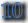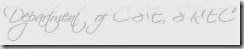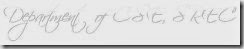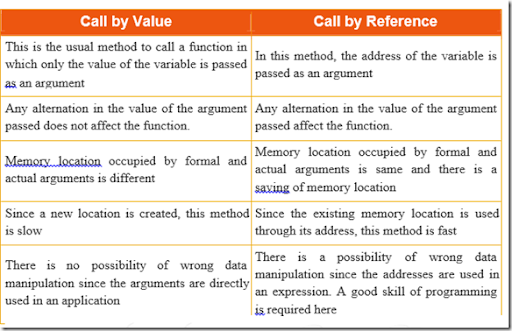### GE6151 Computer Programming 4.2 Parameter Passing Methods/Argument Passing Methods

4.2 Parameter Passing Methods/Argument Passing Methods

Call by Value/Pass by Value:When the value is passed directly to the function it is called call by value. In call by value only a copy of the variable is only passed so any changes made to the variable does not reflects in the calling function.

Example:

#include<stdio.h>

#include<conio.h>

swap(int,int);

void main()

{

int x,y;

printf("Enter two nos");

scanf("%d %d",&x,&y);

printf("\nBefore swapping : x=%d y=%d",x,y);

swap(x,y);

getch();

}swap(int a,int b)

{

int t; t=a; a=b; b=t;

printf("\nAfter swapping :x=%d y=%d",a,b);

}

System Output:

Enter two nos 12 34

Before swapping :12 34

Call by Reference/Pass by Reference:

When the address of the value is passed to the function it is called call by reference. In call by reference since the address of the value is passed any changes made to the value reflects in the calling function.

Example:

#include<stdio.h>

#include<conio.h> swap(int *, int *); void main()

{

int x,y;

printf("Enter two nos");scanf("%d %d",&x,&y);

printf("\nBefore swapping:x=%d y=%d",x,y);

swap(&x,&y);

printf("\nAfter swapping :x=%d y=%d",x,y);

getch();

}

swap(int *a,int *b)

{

int t;

t=*a;

*a=*b;

*b=t;

}

System Output:

Enter two nos 12 34

Before swapping :12 34

Call by Value Call by Reference

This is the usual method to call a function in which only the value of the variable is passed

as an argument

In this method, the address of the variable is passed as an argument

Any alternation in the value of the argument passed does not affect the function.

Memory location occupied by formal and actual arguments is different

Any alternation in the value of the argument passed affect the function.

Memory location occupied by formal and actual arguments is same and there is a saving of memory location

Since a new location is created, this method is slow

There is no possibility of wrong data manipulation since the arguments are directly used in an applicationSince the existing memory location is used through its address, this method is fast

There is a possibility of wrong data manipulation since the addresses are used in an expression. A good skill of programming

is required here